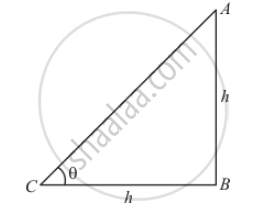Advertisement Remove all ads

# What is the Angle of Elevation of the Sun When the Length of the Shadow of a Vetical Pole is Equal to Its Height? - Mathematics

Sum

What is the angle of elevation of the Sun when the length of the shadow of a vetical pole is equal to its height?

Advertisement Remove all ads

#### Solution

Let C be the angle of elevation of sun is θ.Given that: Height of pole is h meters and length of shadow is h meters. Because length of shadow is equal to the height of pole.

Here we have to find angle of elevation of sun.

So we use trigonometric ratios.

In a triangle ABC,

⇒ tan θ=(AB)/(BC)

⇒ tan θ = h/h

⇒ tan θ=1

⇒θ=45°

Hence the angle of elevation of sun is 45°

Is there an error in this question or solution?
Advertisement Remove all ads

#### APPEARS IN

RD Sharma Class 10 Maths
Chapter 12 Trigonometry
Q 3 | Page 40
Advertisement Remove all ads

#### Video TutorialsVIEW ALL 

Advertisement Remove all ads
Share
Notifications

View all notifications

Forgot password?
Course